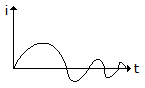# Electronics and Communication Engineering - Networks Analysis and Synthesis

1.

A choke coil of resistance R and inductance L is shunted by capacitor C. The dynamic resistance is

 A. R/LC B. C/RL C. L/RC D. 1/RLC

Explanation:

No answer description available for this question. Let us discuss.

2.

In an LC immittance H (s)

 A. the slope of dH (jω)/dω is always negative B. the slope of dH (jω)/dω is always positive C. the slope of dH (jω)/dω may be positive or negative D. the slope of dH (jω)/dω is constant

Explanation:

No answer description available for this question. Let us discuss.

3.

The response shown in figure isA. over damped B. critically damped C. under damped D. either (a) or (c)

Explanation:

No answer description available for this question. Let us discuss.

4.

The polar plot of P (jω) = 45° as ω varies from -∞ to ∞ is

 A. a straight line parallel to x-axis B. a straight line parallel to y-axis C. a line passing through origin and inclined at 45° D. a line in first quadrant passing through origin and inclined at 45° to positive x-axis

Explanation:

No answer description available for this question. Let us discuss.

5.

Assertion (A): A graph is planar if it has a dual.

Reason (R): The dual of a graph can be found by window dot method.

 A. Both A and R are true and R is correct explanation of A B. Both A and R are true and R is not the correct explanation of A C. A is true but R is false D. A is false but R is true